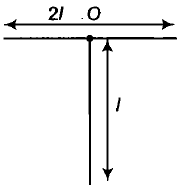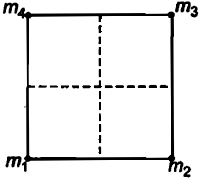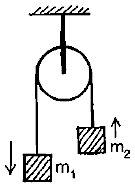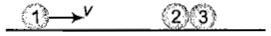A rigid body rotates about a fixed axis with variable angular velocity equal to , at the time t, where $\alpha ,\beta$ are constants. The angle through which it rotates before its stops

1.  $\frac{{\alpha }^{2}}{2\beta }$

2.  $\frac{{\alpha }^{2}-{\beta }^{2}}{2\alpha }$

3.  $\frac{{\alpha }^{2}-{\beta }^{2}}{2\beta }$

4.

Concept Questions :-

Rotation motion : Introduction
High Yielding Test Series + Question Bank - NEET 2020

Difficulty Level:

For the uniform T shaped structure, with mass 3M, the moment of inertia about an axis normal to the plane and passing through O would be1.  $\frac{2}{3}{\mathrm{MI}}^{2}$

2.  ${\mathrm{MI}}^{2}$

3.  $\frac{{\mathrm{MI}}^{2}}{3}$

4.  None of these

Concept Questions :-

Moment of inertia
High Yielding Test Series + Question Bank - NEET 2020

Difficulty Level:

High Yielding Test Series + Question Bank - NEET 2020

Difficulty Level:

An automobile moves on a road with a speed of 54 kmh${}^{-1}$. The radius of its wheel is 0.45 m and moment of inertia of the wheel about its axis of rotation is 3 kg m${}^{2}$. If the vehicle is brought to rest in 15s, the magnitude of average torque transmitted by its brakes to the wheel is

1. 8.58

2. 10.86

3. 2.86

4. 6.66

Concept Questions :-

Torque
High Yielding Test Series + Question Bank - NEET 2020

Difficulty Level:

A rigid body rotates with an angular momentum L. If its rotational kinetic energy is made 4 times, its angular momentum will become

1.  4L

2.  16L

3.

4.  2L

Concept Questions :-

Angular momentum
High Yielding Test Series + Question Bank - NEET 2020

Difficulty Level:

Four particles of mass are placed at four corners of a square. What should be the value of ${\mathrm{m}}_{4}$ so that the center of mass of all the four particles are exactly at the center of the square?1.  2m

2,  8m

3.  6m

4.  None of these

Concept Questions :-

Center of mass
High Yielding Test Series + Question Bank - NEET 2020

Difficulty Level:

Angular momentum of a body is defined as the product of

1.  Mass and angular velocity

3.  Linear velocity and angular velocity

4.  Moment of inertia and angular velocity

Concept Questions :-

Angular momentum
High Yielding Test Series + Question Bank - NEET 2020

Difficulty Level:

Two masses are connected to the ends of massless rope and allowed to move as shown in the figure. The acceleration of the centre of mass assuming pulley is massless and frictionless, is1.

2.  0

3.

4.

Concept Questions :-

Center of mass
High Yielding Test Series + Question Bank - NEET 2020

Difficulty Level:

The speed of a uniform spherical shell after rolling down an inclined plane of vertical height h from rest is

1.

2.

3.

4.

Concept Questions :-

Rolling motion
High Yielding Test Series + Question Bank - NEET 2020

Difficulty Level:

Two identical balls marked 2 and 3. in contact with each other and at rest on a horizontal frictionless table, are hit head-on by another identical ball marked 1 moving initially with a speed v as shown in the figure. Assume the collision is elastic, then mark out the correct statement.1.   Ball 2 comes to rest and ball 2 and 3 moves with speed each

2.  Ball I and 2 come to rest and ball 3 moves with speed v

3   Each ball moves with speed

4.  None of the above

Concept Questions :-

Linear momentum